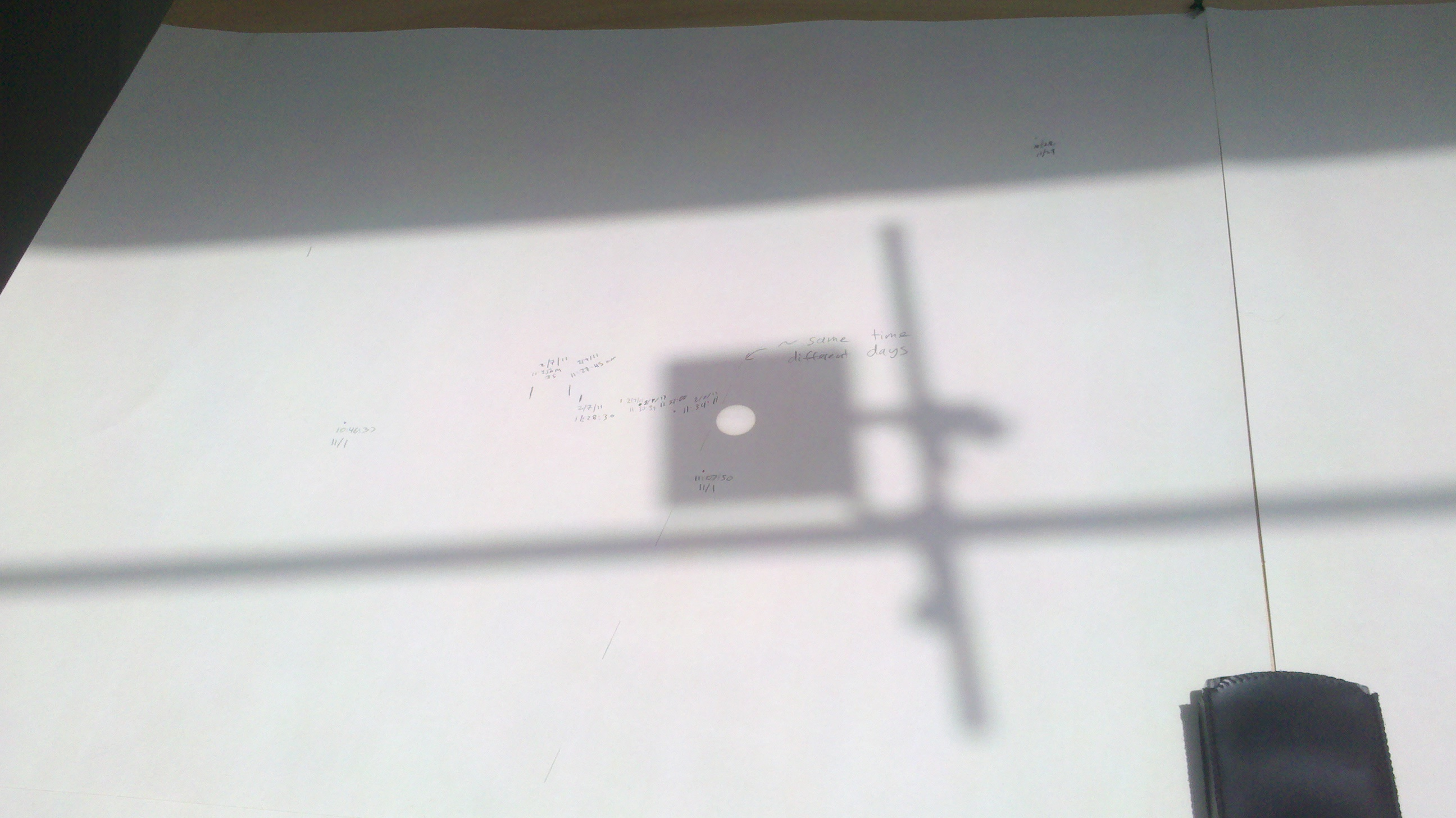Measurement of the Sun's Diameter by Timing its Motion

SPU-21 Fall 2015

How fast does the Sun move across the sky? It appears to move all the way around the Earth (360 degrees) in 24 hours. If we measure how long (minutes and seconds) the Sun takes to move its own diameter along the sundial in our lab, we can measure its angular diameter, in degrees. And from this angle and the known distance to the Sun, the Astronomical Unit (AU), which is approximately 1 AU = 1.5 x 108 km, we can derive the solar diamete, in km.

In the lab you will see a lens attached to the window. The lens is used to focus the Sun's light and make a sharper image on the easel. This is our "sundial".

What you'll need:• Pencil
• A clock that displays seconds
• A sunny day!

Procedure:

• One student should mark the right edge of the projected image of the Sun on the easel and AT THE SAME TIME another student should note the exact time (including seconds).
• Wait until the Sun has moved its entire diameter and as soon as it crosses the mark that you made on the easel, record the new time.
• We suggest each group get 3-5 measurements and then you can take an average and estimate your uncertainty. Enter all numbers in the table (pdf or docx).

Analysis:

• Using simple geometry we will first calculate the angular diameter, θ, of the Sun.
• Seconds in a day/360 degrees = # seconds for sun to cross diameter/ θ, and solve for θ, which will be in degrees
• You can now compute the apparent diameter, D, of the Sun from the "skinny triangle" approximation by finding the small side of the narrow-angle triangle for which you know the long side, d=1AU.
• D = θd, where d = 1AU and θ is the angular diameter that you just calculated (but you need to convert θ to radians first). Note, that the angles in units of radians are defined that 180 degrees = π radians, and so 1 radian = π/180